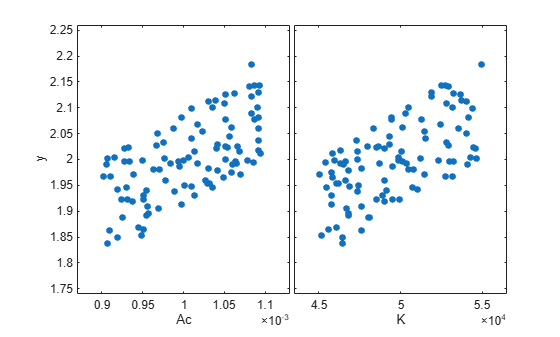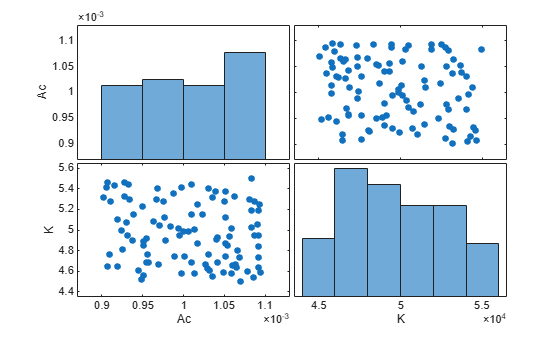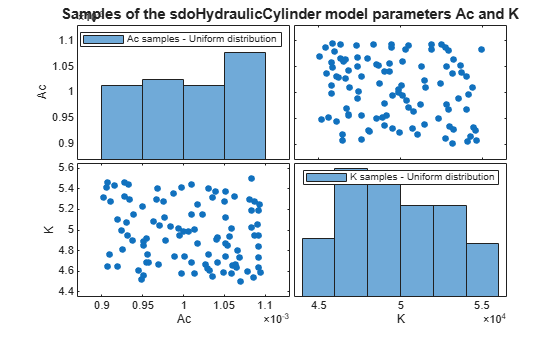sdo.scatterPlot

Scatter plot of samples

Description

example

sdo.scatterPlot(X,Y) creates a matrix of subaxes containing scatter plots of the columns of X against the columns of Y. If X is p-by-n and Y is p-by-m, then sdo.scatterPlot creates a matrix of n-by-m subaxes. X and Y must have the same number of rows.

example

sdo.scatterPlot(X) is the same as sdo.scatterPlot(X,X), except that the subaxes along the diagonal are replaced with histogram plots of the data in the corresponding column of X. For example, the subaxes along the diagonal in the ith column is replaced by hist(X(:,i)).

example

[H,AX,BigAX,P,PAx] = sdo.scatterPlot(___) returns the handles to the graphic objects. Use these handles to customize the scatter plot. For example, you can specify titles for the subaxes.

Examples

collapse all

Generally, you use the sdo.scatterPlot(X,Y) syntax with X specifying the samples and Y specifying the cost function value for each sample. Use the sdo.evaluate command to perform the cost function evaluation to generate Y. For this example, obtain 100 samples of the Ac and K parameters of the sdoHydraulicCyclinder model. Calculate the cost function as a function of Ac and K. Create a scatter plot to see the sample and cost function values.

Generate 100 samples of the Ac and K parameters.

p = sdo.getParameterFromModel('sdoHydraulicCylinder',{'Ac','K'});
ps = sdo.ParameterSpace(p);
X = sdo.sample(ps,100);

The first operation obtains the Ac and K parameters as a vector, p. The second operation creates an sdo.ParameterSpace object, ps, that specifies the probability distributions of the parameter samples. The third operation generates 100 samples of each parameter, returned as a Table, X.

Calculate the cost function value table.

Ac_mean = mean(X{:,1});
K_mean = mean(X{:,2});
Y = table(X{:,1}/Ac_mean+X{:,2}/K_mean,'VariableNames',{'y'});

Create a scatter plot of X and Y.

sdo.scatterPlot(X,Y);Sample the Ac and K parameters of the sdoHydraulicCyclinder model. Use a scatter plot to analyze the samples.

Generate 100 samples of the Ac and K parameters.

p = sdo.getParameterFromModel('sdoHydraulicCylinder',{'Ac','K'});
ps = sdo.ParameterSpace(p);
X = sdo.sample(ps,100);

The first operation obtains the Ac and K parameters as a vector, p. The second operation creates an sdo.ParameterSpace object, ps, that specifies the probability distributions of the parameter samples. The third operation generates 100 samples of each parameter, returned as a Table, X.

Create a scatter plot of X.

sdo.scatterPlot(X);Generate samples of the Ac and K parameters of the sdoHydraulicCyclinder model.

p = sdo.getParameterFromModel('sdoHydraulicCylinder',{'Ac','K'});
ps = sdo.ParameterSpace(p);
X = sdo.sample(ps,100);

Create a scatter plot matrix and return the object handles and the axes handles.

figure
[H,AX,BigAX,P,PAx] = sdo.scatterPlot(X);To set properties for the scatter plots, use the handles in H. To set properties for the histograms, use the patch handles in P. To set axes properties, use the axes handles, Ax, BigAX, and PAx.

Specify a title for the plot matrix and add legends specifying the sample distribution for each parameter.

title('Samples of the sdoHydraulicCylinder model parameters Ac and K')
legend(PAx(1),'Ac samples - Uniform distribution')
legend(PAx(2),'K samples - Uniform distribution')Input Arguments

collapse all

Sampled data, specified as a table.

Cost function evaluation data, specified as a table.

Output Arguments

collapse all

Line object handles, returned as a matrix. This is a unique identifier, which you can use to query and modify the properties of a specific line object. The line objects are used to create the scatter plots.

Subaxes handles, returned as a matrix. This is a unique identifier, which you can use to query and modify the properties of a specific subaxes.

Big axes handle, returned as a scalar. This is a unique identifier, which you can use to query and modify properties of the big axes. BigAX is left as the current axes (gca) so that a subsequent title, xlabel, or ylabel command will center text with respect to the big axes.

Patch object handles, returned as a vector or []. If histogram plots are created, then P is returned as a vector of patch object handles for the histogram plots. These are unique identifiers, which you can use to query and modify the properties of a specific patch object. If no histogram plots are created, then P is returned as empty brackets.

Handle to invisible histogram axes, returned as a vector or []. If histogram plots are created, then PAx is returned as a vector of histogram axes handles. These are unique identifiers, which you can use to query and modify the properties of a specific axes, such as the axes scale. If no histogram plots are created, then PAx is returned as empty brackets.

Introduced in R2014a